## Arithmatical - Heights and Distance

DIRECTIONS : Problems based on Heights and Distance.
1. A ladder learning against a wall makes an angle of 60° with the ground. If the length of the ladder is 19 m, find the distance of the foot of the ladder from the wall. A.   9 m B.   9.5 m C.  10.5 m D.  12 m
Solution
Let AB be the wall and BC be the ladder. and, BC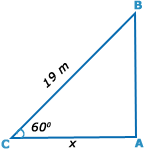Then, < ABC = 60° = 19 m.; AC = x metres AC/BC = cos 60° =1 / 2 x= 19/2 = 9.5 m.
2. The angle of elevation of a ladder leaning against a wall is 60° and the foot of the ladder is 4.6 m away from the wall. The length of the ladder is A.  2.3 m B.  4.6 m C.  7.8 m D.  9.2 m
Solution
Let AB be the wall and BC be the ladder.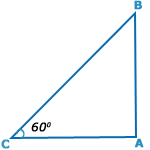Then, < ABC = 60°
AC = 4.6 m.;
AC/BC= cos 60°
= 1 /2
‹=›BC=2×AC
=(2× 4.6) m
= 9.2 m

3. The angle of elevation of the sun, when the length of the shadow of a tree is √3 times the height of the tree is A.  30° B.  45° C.  60° D.  90°
Solution
Let AB be the tree and AC be its shadow.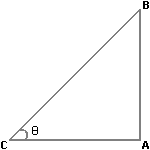Then, < ABC = θ.
Then, AC/AB= √3
cotθ= √3
θ=30°
4. If the height of a pole is 2√3 metres and the length of its shadow is 2 metres, find the angle of elevation of the sun. A.  30° B.  45° C.  60° D.  90°
Solution
Let AB be the pole and AC be its shadow.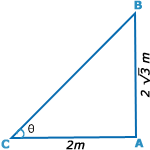Then, < ACB = θ.
Then, AB= 2 √3 m, AC = 2m,
tanθ= AB/AC=2√3/2
= √3
θ=60°
5. From a point P on a level ground, the angle of elevation of the top tower is 30°. If the tower is 100 m high, the distance of point P from the foot of the tower is: A.  149 m B.  156 m C.  173 m D.  200 m
Solution
Let AB be the tower.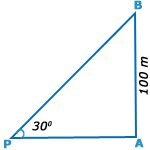Then, <APB =30° and AB= 100 m
AB/AP= tan30°
= 1 / √3
AB = (AB x √3)= 100√3 m.
= (100 x 1.73) m = 173 m.
Page 1 of 2 12